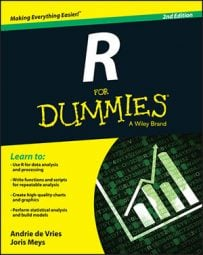##### R For DummiesYou can create a data frame from a matrix in R. Take a look at the number of baskets scored by Granny and her friend Geraldine. If you create a matrix baskets.team with the number of baskets for both ladies, you get this:

```> baskets.team
[,1] [,2] [,3] [,4] [,5] [,6]
baskets.of.Granny   12  4  5  6  9  3
baskets.of.Geraldine  5  4  2  4  12  9```

It makes sense to make this matrix a data frame with two variables: one for Granny’s baskets and one for Geraldine’s baskets.

## Using the function as.data.frame

To convert the matrix baskets.team into a data frame, you use the function as.data.frame():

`> baskets.df <- as.data.frame(t(baskets.team))`

You don’t have to use the transpose function, t(), to create a data frame, but in the example you want each player to be a separate variable. With data frames, each variable is a column, but in the original matrix, the rows represent the baskets for a single player. So, in order to get the desired result, you first have to transpose the matrix with t() before converting the matrix to a data frame with as.data.frame().

## Looking at the structure of a data frame

If you take a look at the object, it looks exactly the same as the transposed matrix t(baskets.team):

```> baskets.df
Granny Geraldine
1st   12     5
2nd   4     4
3rd   5     2
4th   6     4
5th   9    12
6th   3     9```

But there is a very important difference between the two: baskets.df is a data frame. This becomes clear if you take a look at the internal structure of the object, using the str() function:

```> str(baskets.df)
‘data.frame’: 6 obs. of 2 variables:
\$ Granny  : num 12 4 5 6 9 3
\$ Geraldine: num 5 4 2 4 12 9```

Now this starts looking more like a real dataset. You can see in the output that you have six observations and two variables. The variables are called Granny and Geraldine. It’s important to realize that each variable in itself is a vector. In this case, the output tells you that both variables are numeric.

## Counting values and variables

To know how many observations a data frame has, you can use the nrow() function as you would with a matrix, like this:

```> nrow(baskets.df)
 6```

Likewise, the ncol() function gives you the number of variables. But you can also use the length() function to get the number of variables for a data frame:

```> length(baskets.df)
 2```